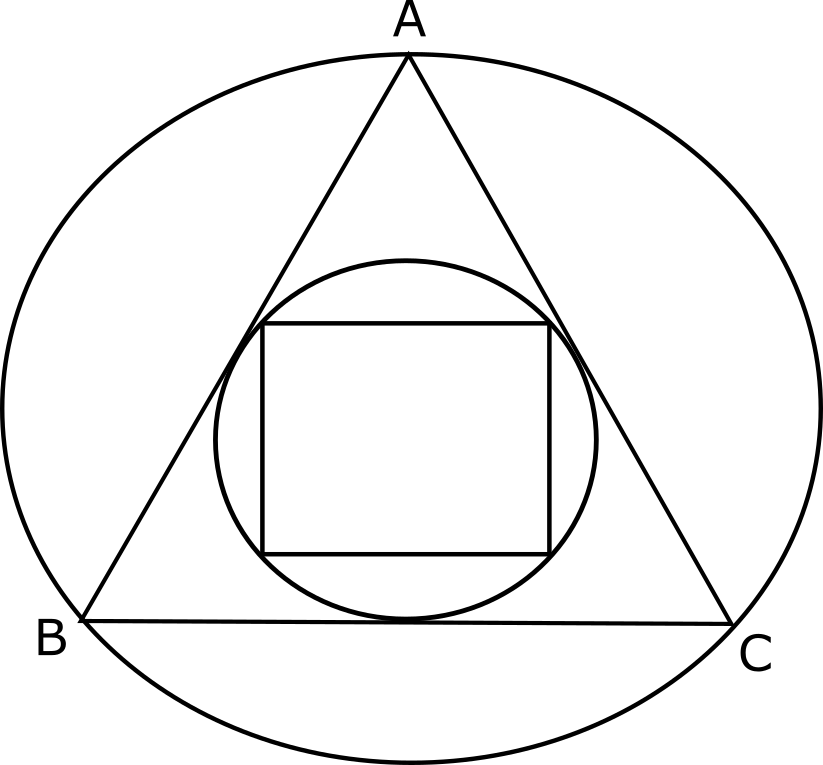## Geometry Test 2

Instructions

For the following questions answer them individually

Q 1

A regular hexagon ABCDEF is inscribed in a unit circle. 3 line segments are drawn connecting the vertex A with C, D, and E. Find the product of lengths of AB, AC, AD, AE and AF.

Q 2

There is a circle of radius 5 cm, in which is inscribed a quadrilateral. It is further known that 2 of the adjacent sides of the quadrilateral are 6 cm and 8 cm. Determine the maximum possible area of the quadrilateral.

Q 3

A square is inscribed in the in-circle of an equilateral triangle ABC as shown. What is the ratio of the area of the square and the area of the circumcircle of the triangle ABC?Q 4

A rectangular swimming pool is 48 m long and 20 m. The shallow edge of the pool is 1 m deep. For every 2.6 m that one walks up the inclined base of the swimming pool, one gains an elevation of 1 m. What is the volume of water (in cubic meters), in the swimming pool? Assume that the pool is filled up to the brim and the inclination is along the length of the pool.

Q 5

From a triangle ABC with sides of lengths 40 ft, 25 ft and 35 ft, a triangular portion GBC is cut off where G is the centroid of ABC. The area, in sq ft, of the remaining portion of triangle ABC is